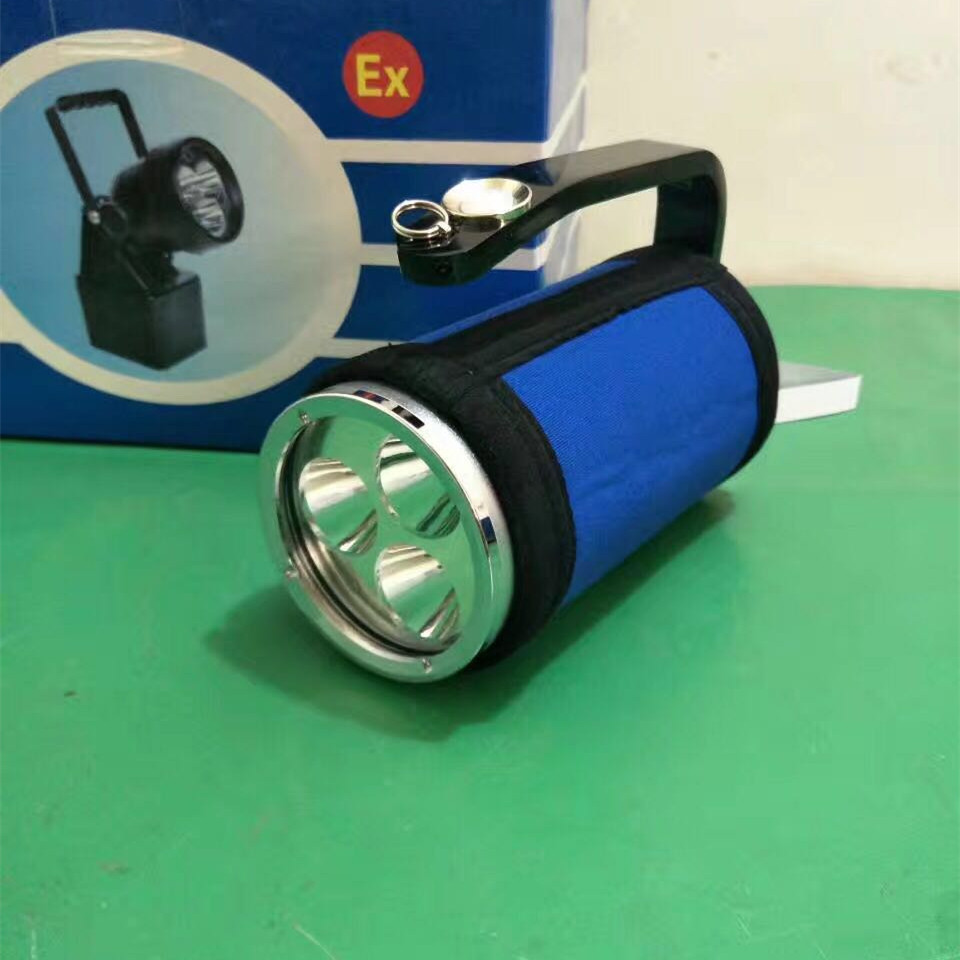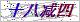• 更新时间：  2019-06-27
• 产品型号：  PD-BB1007
• 简单描述
• PD-BB1007 康庆照明 防爆探照灯 手持肩挎手提灯
PD-BB1007的产品说明信息可能还不够细致和全面，如果您需要更详细了解康庆照明手提强光灯，PD-BB1007的相关信息或索取相关资料，欢迎随时与我！康庆照明客服：小周

PD-BB1007 康庆照明 防爆探照灯 手持肩挎手提灯 RJW7101/LT厂家防爆证

^^^^^^^^^^^^^^^PD-BB1007^^^^^^^^^^^^^^^^^^^^

^^^^^^^^^^^^^^^PD-BB1007^^^^^^^^^^^^^^^^^^^^

^^^^^^^^^^^^^^^PD-BB1007^^^^^^^^^^^^^^^^^^^^

^^^^^^^^^^^^^^^PD-BB1007^^^^^^^^^^^^^^^^^^^^

1.灯光 控 制：一次轻按开关按钮，强光工作模式；二次轻按开关按钮，转换为工作光工作模式；第三次轻按开关按钮，电源关闭。

2.信号光控制：无论电源启动或关闭，长按开关按钮5秒左右，灯具进入信号指示模式；再次按压开关按钮，电源关闭。

PD-BB1007 康庆照明 防爆探照灯 手持肩挎手提灯 RJW7101/LT厂家防爆证PD-BB1007的产品说明信息可能还不够细致和全面，如果您需要更详细了解康庆照明手提强光灯，PD-BB1007的相关信息或索取相关资料，欢迎随时与我！康庆照明客服：小周

### 留言框

• #### 验证码：请输入计算结果（填写阿拉伯数字），如：三加四=7

13616875157